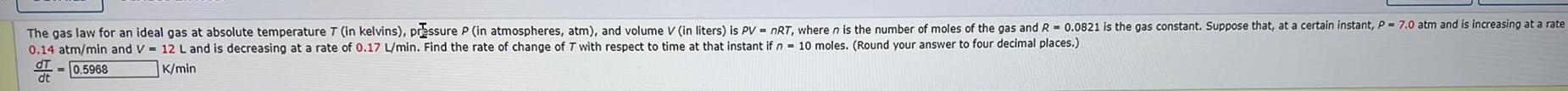Question:

# The gas law for an ideal gas at absolute temperature 7 (in

Last updated: 7/8/2022The gas law for an ideal gas at absolute temperature 7 (in kelvins), pressure P (in atmospheres, atm), and volume V (in liters) is PV = nRT, where n is the number of moles of the gas and R = 0.0821 is the gas constant. Suppose that, at a certain instant, P = 7.0 atm and is increasing at a rate 0.14 atm/min and V 12 L and is decreasing at a rate of 0.17 L/min. Find the rate of change of T with respect to time at that instant if n 10 moles. (Round your answer to four decimal places.) dT/dt = ______K/min